# ASM 100: x86 Assembly Code (69 pts)

## What You Need

• A Google Cloud Debian 10 Linux Server

## Purpose

To practice writing simple 32-bit assembly code.

## Installing Tools

We'll need a few tools. On your Linux server, in an SSH window, execute these commands:
```sudo apt update sudo apt install build-essential gcc-multilib gdb nasm netcat -y ```

## Hello, World in Assembler

First we'll get a simple program working without worrying about details. There will be a detailed explanation of the code later.

Execute this command to create a "hello.asm" file:

```nano hello.asm ```
Enter this code, as shown below:
```section .text global _start _start: mov edx, len mov ecx, msg mov ebx, 1 mov eax, 4 int 0x80 mov eax, 1 int 0x80 section .data msg db "Hello World!" len equ \$ - msg ```
Press Ctrl+X, Y, Enter to save the file/Execute these commands to compile, link, and run the program:

```nasm -f elf32 hello.asm ld -m elf_i386 -o hello hello.o ./hello ```
The program runs, as shown below.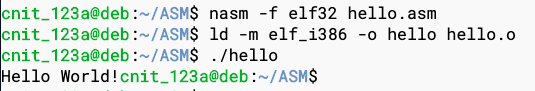## Explanation

Note these features of the assembly program you just made:
• The two sections: .text and .data
• The _start label telling the compiler where the executable commands begin
• The int 0x80 commands: these "system calls" execute kernel functions

## System Calls

The table below shows the first four Linux System Calls, from this page.

The eax register defines which system call you are making, and the other registers are used for parameters, as shown below.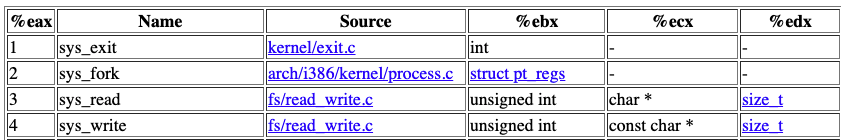## Syscall #4: sys_write

The program above used the code below to write to the console. The Syscall number in eax is 4, so this is a call to sys_write.

Here's the prototype of system call 4, from this page: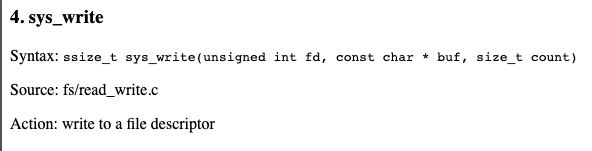Here's that code with explanatory comments:

``` mov edx, len ; length of string to print mov ecx, msg ; address of string to print mov ebx, 1 ; file descriptor: 1 = stdout mov eax, 4 ; syscall #4 is sys_write int 0x80 ; execute the syscall ```

## Syscall #1: sys_exit

Here's the prototype of system call 1, from this page:Here's that code with explanatory comments:

``` mov eax, 1 ; syscall #1 is sys_exit int 0x80 ; execute the syscall ```

## .data Section

The data section defines two variables, as shown below.
```section .data msg db "Hello World!" ; db = Define Byte, ASCII encoded len equ \$ - msg ; defines a constant ```
The "len" constant is calculated by subtracting the address of the "msg" variable from the address of "len" itself.

## Theory

If you just want to do the hands-on projects, skip this section.

## Background

Refer to this tutorial:
Assembly Programming Tutorial
Answer the questions below.

## ASM 100.1: Section (2 pts)

There are three sections to an assembly program, including the text and data sections used above. What is the third section?

## ASM 100.2: Size (2 pts)

How many bits are in the AX register?

## ASM 100.3: Syscall (2 pts)

What's the name of system call #6?

## ASM 100.4: TBYTE (2 pts)

How many bytes are in a TBYTE?

## ASM 100.5: MUL (2 pts)

When two DWORD values are multiplied, what register contains the higher-order bits?

## ASM 100.6: XOR (2 pts)

What is the value of eax after processing these commands?
``` mov eax, 19 xor eax, 61 ```

## ASM 100.7: NOT (2 pts)

What is the value of eax after processing these commands?
``` mov eax, 19 not eax ```

## ASM 100.8: LOOP (2 pts)

Where is the loop count stored when using the LOOP instruction?

## ASM 100.9: CMPSD (2 pts)

What register points to the destination operand when using the CMPSD instruction?

## ASM 100.10: REP (2 pts)

What register controls the number of repitions when using the REP prefix?

## ASM 100.11: '6' (2 pts)

What is the decimal value in EBX after this instruction is executed?
``` mov ebx, '6' ```

## ASM 100.12: 01000001B (2 pts)

What is the decimal value in EBX after this instruction is executed?
``` mov ebx, 01000001B ```

## ASM 100.13: sys_write (5 pts)

What is the decimal value in EAX after this code is executed?
``` mov BYTE [msg], 48 mov edx, 6 mov ecx, msg mov ebx, 1 mov eax, 4 int 0x80 ```

## ASM 100.14: pop (2 pts)

What is the decimal value in EAX after this code is executed?
``` push 33H push 108 pop eax pop eax ```

## ASM 100.15: (3 pts)

What is the decimal value in EDX after the instruction outlined in green below is executed?## ASM 100.16: HEAD (5 pts)

Write a program named head that constructs a HEAD request, as shown below.

Then execute this command to get the flag, which is covered by a green rectangle in the image below:

```./head | nc ad.samsclass.info 80 ```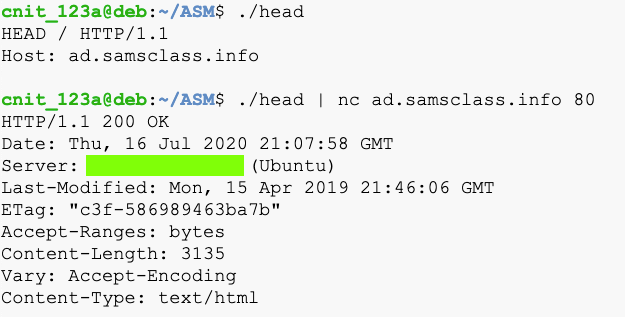Hint: Each line must end with a carriage return and a line feed, in that order.

## ASM 100.17: GET (5 pts)

Open this Web page:

http://target1.bowneconsulting.com/php/asm1.phpYou don't get the flag.

Write a program named get that constructs the correct a GET request, as shown below.

Then execute this command to get the flag, which is covered by a green rectangle in the image below:

```./get | nc target1.bowneconsulting.com 80 ```## ASM 100.18: BRUTE (10 pts)

Open this Web page:

http://target1.bowneconsulting.com/php/asm2.php

Write a program that logs in with these parameters, as shown below:

• HTTP method is GET, as in the previous challenge
• Password: password followed by a single digit
• User-Agent: ASMCODE
Hint: use a loop.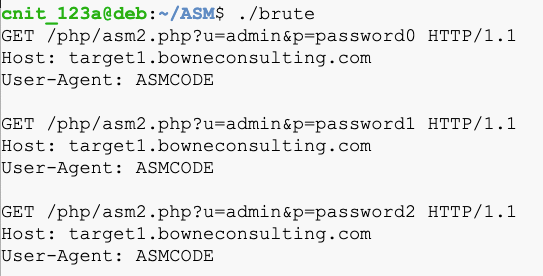The flag is covered by a green rectangle in the image below:## ASM 100.19: BRUTE (15 pts)

Open this Web page:

http://target1.bowneconsulting.com/php/asm3.php

Write a program that logs in with these parameters, as shown below:

• HTTP method is GET, as in the previous challenge
• Username: admin followed by a single digit
• Password: password followed by a single digit
• User-Agent: ASMCODE
A successful login will reveal the flag.

## Sources

System calls in the Linux kernel. Part 1.
X86 Assembly/Interfacing with Linux
Linux System Call Table (32-bit)
List of Linux/i386 system calls
Assembly Programming Tutorial

Posted 7-16-2020
Hint added to ASM 100.18 7-17-2020